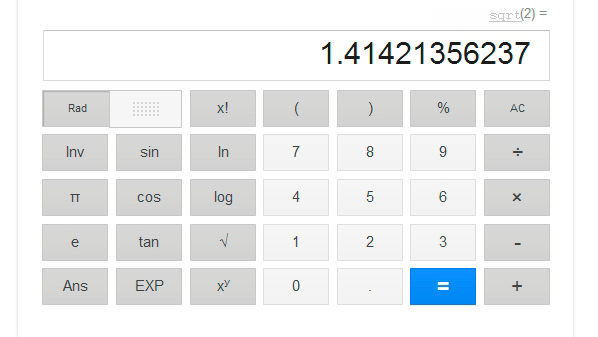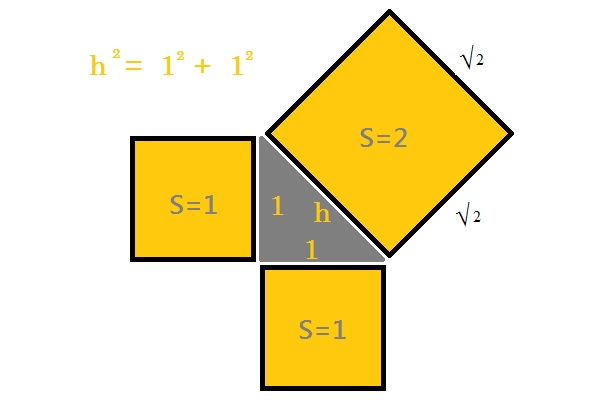# Square root of 2

We know how we can calculate by hand the square root, and we also know that the result of the square root of a number X is other number whose square is X. Then, the square root of 2 is the real number whose square is 2.The importance of this issue comes when we apply the Pythagorean Theorem to a square of size 1 by 1. The value of the hypotenuse is determined by the formula:$h^2=1^2+1^2$

That is:$h=\sqrt{2}$

This makes the square root of 2, possibly the first irrational number employee in the history of mathematics, remember that an irrational number is any real number that can not be expressed as a fraction m / n.As curious to note from wikipedia, without going into concepts that are beyond the middle school level discussed in this blog, is that its value has been calculated up to the incredible number of 137 438 953 444 decimal places by Yasumasa Kanada’s team in 1997, but, In February 2006 this record was eclipsed with the use of a home computer (200,000,000,000 decimal places). This makes him the second number, after π, where more decimals has been calculated.$\sqrt{2}=1,4142135623730950488016887242097\dots$